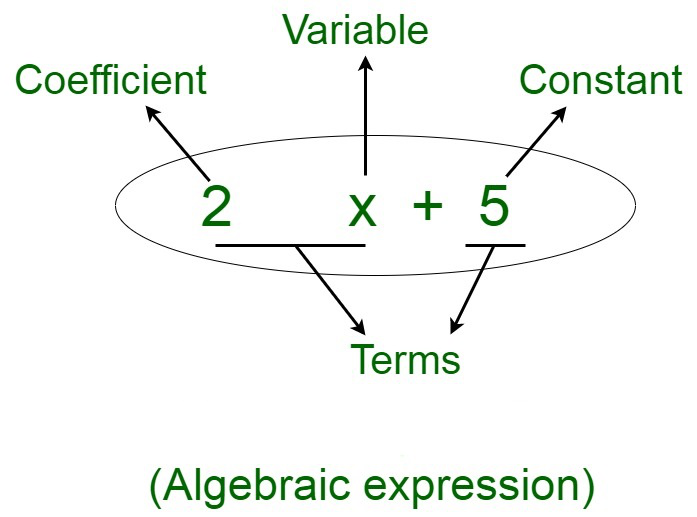GeeksforGeeks App
Open AppBrowser
Continue

# How many terms are there in an algebraic expression?

An algebraic expression is an expression composed of various components, such as variables, constants, coefficients, and arithmetic operations. These components form various parts of the algebraic expressions. An algebraic expression is a linear equation composed of any number of variables. The highest power of the variable is known as the degree. An algebraic expression containing one variable is monomial, two variables is binomial, and so on. For instance, if we assume an expression to be,

2x+5

Here, the parts of the expression are as follows:

Coefficient of the expression is 2

Constant is 5

Variable is x

Terms are 2x and 5

Mathematical operators used are plus (+) and minus (-).### What are Terms?

The terms of an algebraic expression are known as the components of the expression. An algebraic expression may be composed of one or more terms. A term of an expression may be a constant, a variable, a product of more than two variables (xy), or a product of a variable and a constant. Terms add up together to form an algebraic expression. For instance, in the algebraic expression 3x + y, the two terms are 3x and y. Terms can be further classified depending on the variables and the corresponding powers defining them. They may be divided into like and unlike terms:

• Like terms: The terms which are constituted by the same literal along with the same exponents. For example: 12x and -3x are like terms since both of them have the same power of the same variable.
• Unlike terms: The terms which are constituted by the same variable with different exponents or different variables with the same exponents. For example: x and x2 are unlike terms.

An algebraic expression can be composed of the following terms:

Coefficient

A coefficient is an integer that is the constant which accompanies the variable. To summarise, a coefficient in an algebraic expression is considered as the numerical factor of a term that is composed of constants and variables. Coefficients of the terms may be positive or negative in nature. They may be fractional in nature. For instance, in the term 2x, 2 is the coefficient for the variable x. The terms with no constant, that is with no numerical factor along with them have a unit coefficient. For instance, in the term z, +1 is the coefficient for the variable z. Similarly, we have, -5/2 as the coefficient of the term –5/2xy2

Constant

Constant is the term in the algebraic expression which is constituted of only integers. These terms contain variable counterparts. These values are fixed in nature since there is no variable accompanying them. Therefore, these terms have a fixed value throughout, since no change can occur in these. Terms may only be defined by constants. For instance, in the expression 7x2 + 3xy + 8, the constant term in this expression is 8.

Variable

Variables are terms composed of undefined values, which may assume different integer values on substituting them with different integers. A variable term can be composed of one or more variables, where the variables may or may not be the same. For example, we have, x3 which is a term composed of x raised to the power of 3, and xyz is composed of three different variables.

For instance, x3 can be 8 where the value of x = 2.

Some of the examples of terms are:

• 12x: Constant term = 12 ; Variable term = x
• 42a: Constant = 42 ; Variable = a
• xy: Variables = x and y
• 89: Constant = 89
• mn: Constant = 1 ; Variables = m and n

### Sample Questions

Question 1. Identify the terms, like terms, coefficients, and constants in the expression.

12m − 24n + 10 + m − 17

Solution:

Here, we have,

12m − 24n + 10 + m − 17

First, rewrite the subtractions as additions.

12m − 24n + 10 + m − 17 = 12m + (-24n) + 10 + m + (-17)

Therefore,

The terms: 12m, (−24n), m, 10, and (−17).

The like terms are the ones that contain the same variable

12m and m are a pair of like terms.

The constant terms 10 and −17 are like terms.

Coefficients: 12 is coefficient of m,

-24 is the coefficient of n

1 is the coefficient of m.

Therefore, the coefficients are 12, (−24), and 1.

Constants: 10, -17

Question 2. Differentiate between constants and variables.

Solution:

Question 3. Compute the value of x in the equation 2x + 20 = 40

Solution:

Given,

2x + 20 = 40

⇒ 2x + 20 = 40

⇒ 2x = 40 – 20

⇒ 2x = 20

⇒ x =⇒ x = 10

Value of x in the equation 2x + 20 = 40 is 10.

Question 4. Find the Variable, coefficient, constant, and terms of the algebraic expression

90x + 22y – 31

Solution:

Here given algebraic expression

90x + 22y – 31

We have to find  Variable, coefficient, constant, and terms

Variables: x and y

Terms: 90x, 22y and 31

Constant: 31

Coefficient: 90 of x and 22 of y.

My Personal Notes arrow_drop_up
Related Tutorials